Browse by Topic
Related Topics

# The Spoons and the Summer School

During my stay in Champaign, Illinois at Wolfram Research headquarters last summer, I attended the 2008 Advanced Mathematica Summer School. The Summer School gives people from all over the world a chance to present their challenging problems in varied math and science fields and work with others to find solutions using Mathematica. These research topics cover a very wide range of application areas. My personal interest is in numerics, and I had the chance to work on several related projects.

While at the Summer School, one of the projects I was involved in was to analytically derive a mechanism to compute the stress distribution in a circular plate with concentrated radial loadings.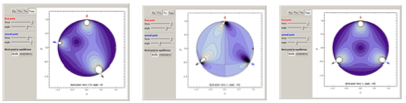Related to that, we investigated the stress induced by a crack with the finite element method. Below we have a block with a crack. The block is constrained on the left, and forces are applied on the top and bottom to tear the block.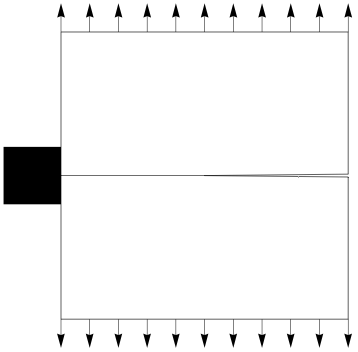The upper part of the block has different material properties than the lower part. In the lower part, the Young’s modulus is half that of the upper part. I modeled this as a plain-stress, coupled partial differential equation. To solve the model, I used the finite element method. First, I created an input file for the Triangle mesh generator. Next, I wrote the operators that actually computed the contribution from each finite element, and then assembled those elements into a system of equations. For this case, I used 12,000 quadratic elements. The final system of equations had a little more than 52,000 degrees of freedom. Here is an exaggerated displacement of the mesh.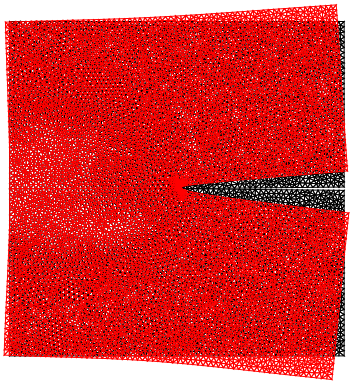Then I computed the stress distribution in the block, which is depicted below.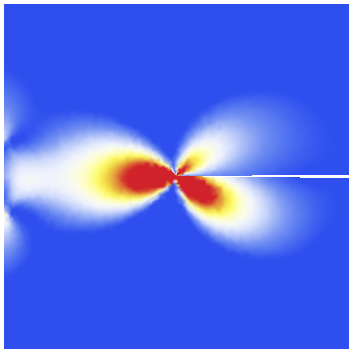I had a chance to discuss partial differential equations and the finite element method with Stephen Wolfram when we happened to be seated next to each other at the Summer School dinner. He has a very inquiring mind, and at one point he looked around the table and the object that caught his eye was a spoon. “So, Oliver, how many degrees of freedom would be needed to model a spoon and be able to manipulate it?” he asked. Because the spoon has a high aspect ratio—it is long and flat—my guess was that about 50,000 degrees of freedom were needed. But I was not sure if this was a reasonable estimate, so I downloaded a 3Ds spoon model from 3dsmodels.com.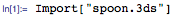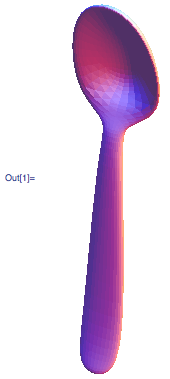With some old code I had written, I converted the 3D model into an input file for the TetGen mesh generator. That resulted in a mesh with a little more than 12,000 elements and some 3,000 nodes. Below you see the bowl of the spoon and the mesh.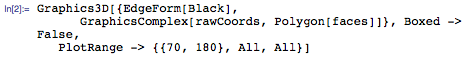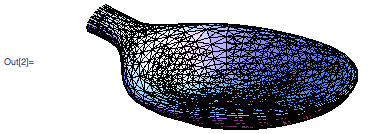The bending of the spoon is a structural mechanics problem. It can also be viewed as a coupled partial differential equation. In this setting, each node has three degrees of freedom—in the x, y, and z directions.

The next step was to compute the contribution from each element to the system of equations. All in all, that resulted in a system of equations with a total of about 9,000 degrees of freedom.

Once I had the system of equations assembled, the boundary conditions should have reflected the constraints that the spoon is held fixed at one end and that the tip at the other end is bent. The bending is done through a force applied to the tip of the bowl.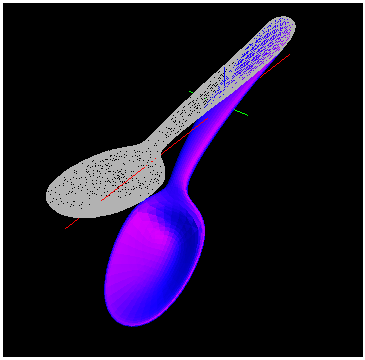So my initial guess that 50,000 degrees of freedom were needed was a little too high. 10,000 degrees of freedom did a reasonable job.

The Advanced Mathematica Summer School 2009 is almost here. I can’t wait to see what projects the students complete this year!